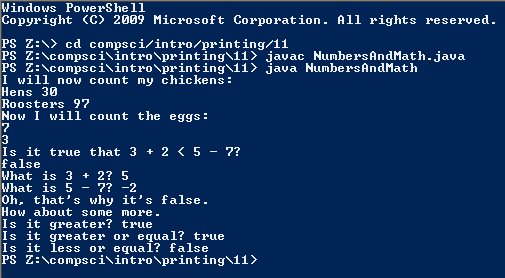# Assignemnt #11 and Numbers And Math

## Code

```      ///Name: Dakota Donahue
///Period: 5
///Project Name: Numbers And Math
///File Name: NumbersAndMath
///Date: 9/9/2015

public class NumbersAndMath
{
public static void main( String[] args )
{
System.out.println( "I will now count my chickens:" );
System.out.println( "Hens " + ( 25 + 30 / 6 ) );
//Subtracting the product of 25 and (3 % 4) from 100
System.out.println( "Roosters " + ( 100 - 25 * 3 % 4 ) );
System.out.println( "Now I will count the eggs:" );
//Adds 3,2,1,-5,0 (4 % 2),-1/4, and 6
System.out.println( 3 + 2 + 1 - 5 + 4 % 2 - 1 / 4.0 + 6.0 );
System.out.println( "Is it true that 3 + 2 < 5 - 7?" );
//Determining if the sum of 3 and 2 is less than the difference of 5 and 7
System.out.println( 3 + 2 < 5 - 7 );
System.out.println( "What is 3 + 2? " + ( 3 + 2 ) );
//Subtracting 7 from 5
System.out.println( "What is 5 - 7? " + ( 5 - 7 ) );
System.out.println( "Oh, that's why it's false." );
System.out.println( "How about some more." );
//Determining if 5 is larger than -2
System.out.println( "Is it greater? " + ( 5 > -2 ) );
//Determining if 5 is greater than or equal to -2
System.out.println( "Is it greater or equal? " + ( 5 >= -2 ) );
//Determining if 5 is less than or equal to -2
System.out.println( "Is it less or equal? " + ( 5 <= -2 ) );
}
}
```

### Picture of the output